Function Repository Resource:

# TuringMachineToNumber

Convert an explicit list of rules into an enumerated Turing machine specification

Contributed by: Mano Namuduri
 ResourceFunction["TuringMachineToNumber"][rules] converts rules to an enumerated Turing machine specification containing the rule number, number of states and number of colors.

## Details

The input rules should be a list of Rule or RuleDelayed elements of the form {si, ai} -> {sf, af, offset}, each of which contains Integer elements denoting the following:
 si initial state of the head ai initial value of the cell under the head sf final state of the head af final value of the cell under the head offset offset by which the head moves
The output of ResourceFunction["TuringMachineToNumber"] is of the form {n, s, k}, with integer elements denoting the following:
 n number encoding the given rules s number of states in the Turing machine k number of tape cell colors in the Turing machine
The number of possible Turing machine rules is as follows:
 2-state, 2-color machines 4096 s-state, k-color machines (2sk)^(sk)

## Examples

### Basic Examples (2)

Convert a list of Turing machine rules into an enumerated specification:

 In:=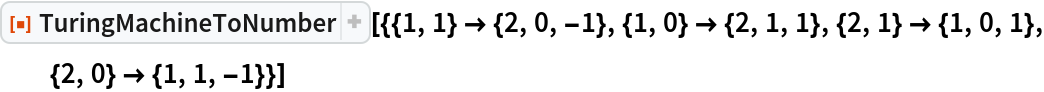Out=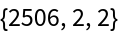Show that the two specifications are equivalent:

 In:=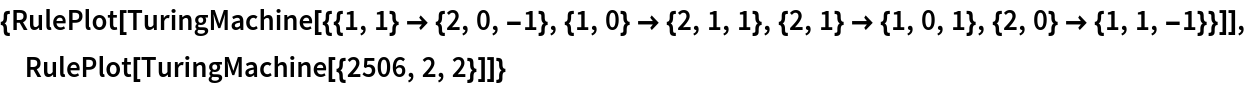Out=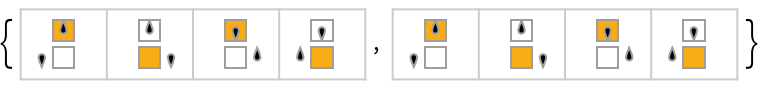### Scope (2)

Generate all possible rules for a 1-state, 2-color Turing machine, ordered by rule number:

 In:=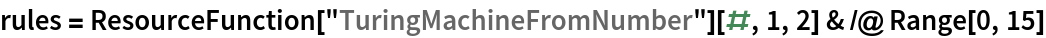Out=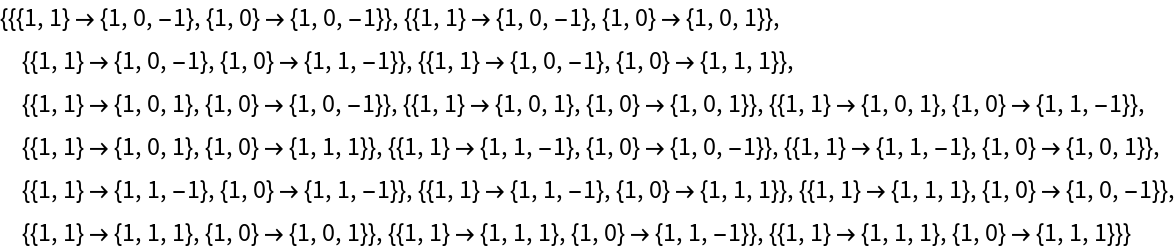Convert the set of possible s = 1, k = 2 rules back to enumerated form:

 In:=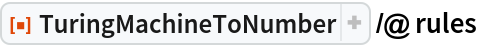Out=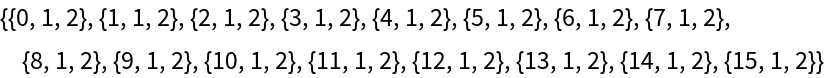### Possible Issues (3)

In a valid Turing machine specification, head states must be integers greater than 0:

 In:=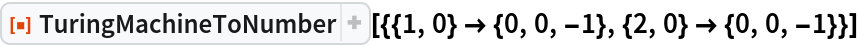Out=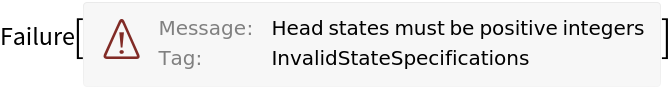In a valid Turing machine specification, tape colors must be integers greater than or equal to 0:

 In:=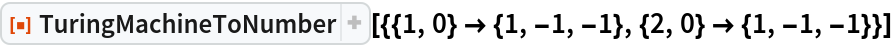Out=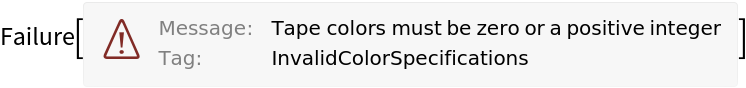TuringMachineToNumber cannot accept offsets with the head List:

 In:=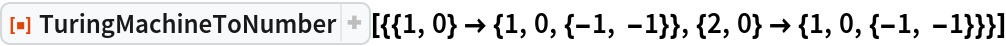Out=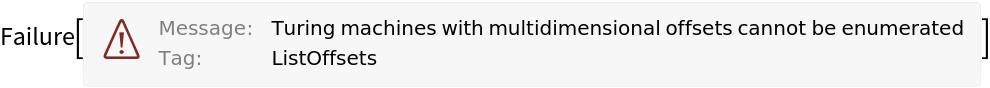TuringMachineToNumber cannot accept offsets that are not 1 or -1:

 In:=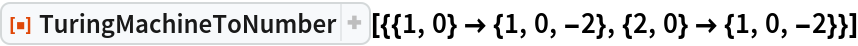Out=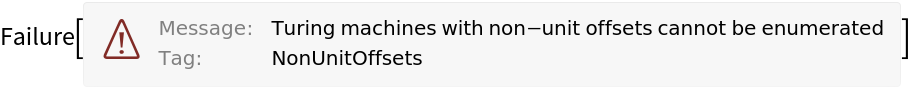Turing machines that have multiple transformations for a single input configuration cannot be enumerated:

 In:=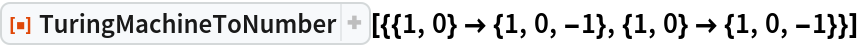Out=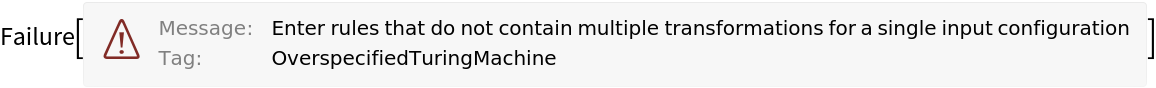Turing machines that do not have a transformation for every possible input configuration cannot be enumerated:

 In:=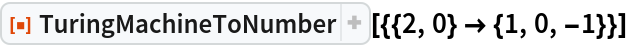Out=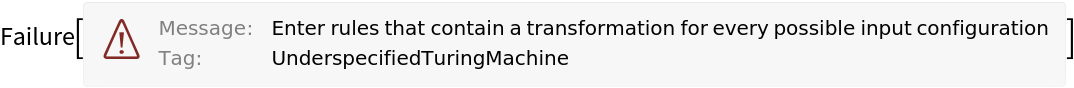## Version History

• 1.0.0 – 12 April 2021

## Author Notes

In the future, this function could be extended to accept non-unit offsets. In this case, it could return the rule format {n, s, k, {{off1}, {off2}, …}}, which explicitly gives the offset for each rule and is a format currently accepted by TuringMachine.